Tousled Tangerine Blur
December 26, 2016

# Tousled Tangerine Blur

VIEW ALL PHOTOS VIEW SLIDESHOW
GET THE HOW-TO• Formula A

z.one concept milk_shake smoothies conditioning semi permanent color 6.43 + z.one activating emulsion

• Formula B

milk_shake smoothies conditioning semi permanent color 6.6 + activating emulsion

• Formula C

milk_shake smoothies conditioning semi permanent color 7.43 + 8.43 + activating emulsion

• Formula D

z.one lighblue dust-free conditioning powder bleach + 10-volume (3%) developer# Tousled Tangerine Blur

This quick and easy color placement technique from the z.one concept Red Collection involves square and triangular sections which allow you to create “hidden color” that can be displayed or concealed. The color palette combines bright and rich copper tones, but that’s just the beginning! This technique can be done with any color combination from palest blonde to deep brunette and beyond.

Artist: Elisa Vidotto
Photographer: Kamil Struzinski
Makeup Artist: Martina D'Andrea

## COLOR FORMULAS• Formula A

z.one concept milk_shake smoothies conditioning semi permanent color 6.43 + z.one activating emulsion

• Formula B

milk_shake smoothies conditioning semi permanent color 6.6 + activating emulsion

• Formula C

milk_shake smoothies conditioning semi permanent color 7.43 + 8.43 + activating emulsion

• Formula D

z.one lighblue dust-free conditioning powder bleach + 10-volume (3%) developer

## HOW-TO STEPS•• Formula A

z.one concept milk_shake smoothies conditioning semi permanent color 6.43 + z.one activating emulsion

• Formula B

milk_shake smoothies conditioning semi permanent color 6.6 + activating emulsion

• Formula C

milk_shake smoothies conditioning semi permanent color 7.43 + 8.43 + activating emulsion

• Formula D

z.one lighblue dust-free conditioning powder bleach + 10-volume (3%) developer

1

Create a square section at the crown, then create four triangular sections off of each side of the square.

•• Formula A

z.one concept milk_shake smoothies conditioning semi permanent color 6.43 + z.one activating emulsion

• Formula B

milk_shake smoothies conditioning semi permanent color 6.6 + activating emulsion

• Formula C

milk_shake smoothies conditioning semi permanent color 7.43 + 8.43 + activating emulsion

• Formula D

z.one lighblue dust-free conditioning powder bleach + 10-volume (3%) developer

2

Completed sections.

•• Formula A

z.one concept milk_shake smoothies conditioning semi permanent color 6.43 + z.one activating emulsion

• Formula B

milk_shake smoothies conditioning semi permanent color 6.6 + activating emulsion

• Formula C

milk_shake smoothies conditioning semi permanent color 7.43 + 8.43 + activating emulsion

• Formula D

z.one lighblue dust-free conditioning powder bleach + 10-volume (3%) developer

3

Apply Formula A to the regrowth area in the back section.

•• Formula A

z.one concept milk_shake smoothies conditioning semi permanent color 6.43 + z.one activating emulsion

• Formula B

milk_shake smoothies conditioning semi permanent color 6.6 + activating emulsion

• Formula C

milk_shake smoothies conditioning semi permanent color 7.43 + 8.43 + activating emulsion

• Formula D

z.one lighblue dust-free conditioning powder bleach + 10-volume (3%) developer

4

Continue applying Formula A to the remaining lengths and ends.

•• Formula A

z.one concept milk_shake smoothies conditioning semi permanent color 6.43 + z.one activating emulsion

• Formula B

milk_shake smoothies conditioning semi permanent color 6.6 + activating emulsion

• Formula C

milk_shake smoothies conditioning semi permanent color 7.43 + 8.43 + activating emulsion

• Formula D

z.one lighblue dust-free conditioning powder bleach + 10-volume (3%) developer

5

Completed application.

•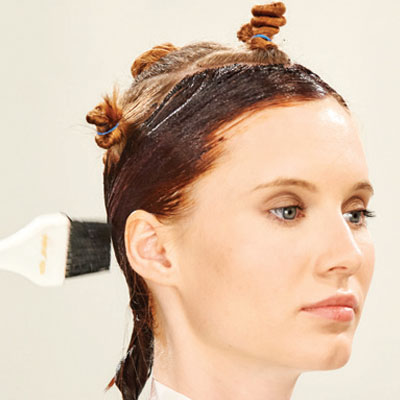• Formula A

z.one concept milk_shake smoothies conditioning semi permanent color 6.43 + z.one activating emulsion

• Formula B

milk_shake smoothies conditioning semi permanent color 6.6 + activating emulsion

• Formula C

milk_shake smoothies conditioning semi permanent color 7.43 + 8.43 + activating emulsion

• Formula D

z.one lighblue dust-free conditioning powder bleach + 10-volume (3%) developer

6

Apply Formula A to the remaining hair that is outside the square and triangular sections.

•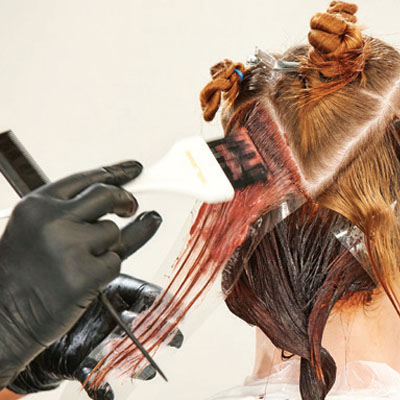• Formula A

z.one concept milk_shake smoothies conditioning semi permanent color 6.43 + z.one activating emulsion

• Formula B

milk_shake smoothies conditioning semi permanent color 6.6 + activating emulsion

• Formula C

milk_shake smoothies conditioning semi permanent color 7.43 + 8.43 + activating emulsion

• Formula D

z.one lighblue dust-free conditioning powder bleach + 10-volume (3%) developer

7

Create two fine sections on the outer perimeter of the rear triangle and apply Formula B from roots to ends.

•• Formula A

z.one concept milk_shake smoothies conditioning semi permanent color 6.43 + z.one activating emulsion

• Formula B

milk_shake smoothies conditioning semi permanent color 6.6 + activating emulsion

• Formula C

milk_shake smoothies conditioning semi permanent color 7.43 + 8.43 + activating emulsion

• Formula D

z.one lighblue dust-free conditioning powder bleach + 10-volume (3%) developer

8

Working in fine sections, repeat this application in the remaining three triangular sections.

•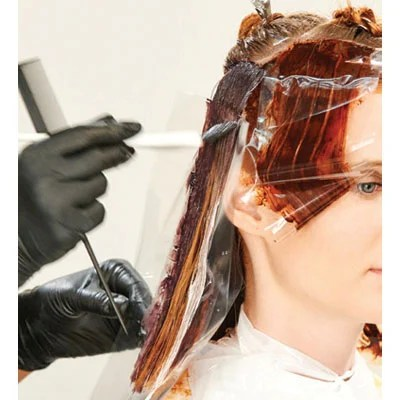• Formula A

z.one concept milk_shake smoothies conditioning semi permanent color 6.43 + z.one activating emulsion

• Formula B

milk_shake smoothies conditioning semi permanent color 6.6 + activating emulsion

• Formula C

milk_shake smoothies conditioning semi permanent color 7.43 + 8.43 + activating emulsion

• Formula D

z.one lighblue dust-free conditioning powder bleach + 10-volume (3%) developer

9

Create a horizontal parting at the edge of each triangle and apply Formula A roots to ends.

•• Formula A

z.one concept milk_shake smoothies conditioning semi permanent color 6.43 + z.one activating emulsion

• Formula B

milk_shake smoothies conditioning semi permanent color 6.6 + activating emulsion

• Formula C

milk_shake smoothies conditioning semi permanent color 7.43 + 8.43 + activating emulsion

• Formula D

z.one lighblue dust-free conditioning powder bleach + 10-volume (3%) developer

10

Create a second horizontal parting at the edge of each triangle. Apply Formula C to the left side and Formula D to the right side, blending the two formulas with a clean brush.

•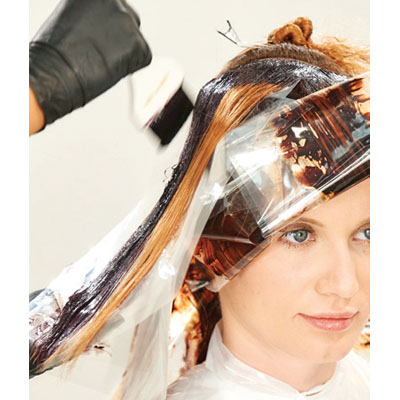• Formula A

z.one concept milk_shake smoothies conditioning semi permanent color 6.43 + z.one activating emulsion

• Formula B

milk_shake smoothies conditioning semi permanent color 6.6 + activating emulsion

• Formula C

milk_shake smoothies conditioning semi permanent color 7.43 + 8.43 + activating emulsion

• Formula D

z.one lighblue dust-free conditioning powder bleach + 10-volume (3%) developer

11

Repeat step 10 on a third horizontal subsection of each triangle, but this time, alternate the color placement.

•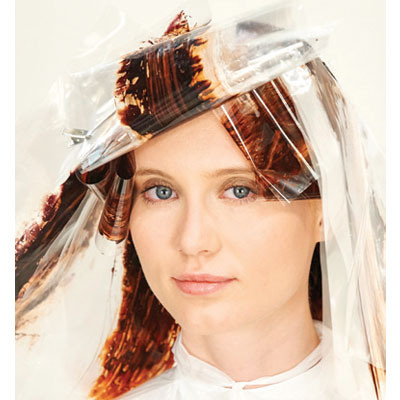• Formula A

z.one concept milk_shake smoothies conditioning semi permanent color 6.43 + z.one activating emulsion

• Formula B

milk_shake smoothies conditioning semi permanent color 6.6 + activating emulsion

• Formula C

milk_shake smoothies conditioning semi permanent color 7.43 + 8.43 + activating emulsion

• Formula D

z.one lighblue dust-free conditioning powder bleach + 10-volume (3%) developer

12

Divide the square section with a diagonal parting. Apply Formula C to one side. On the other side, continue the application technique from steps 10 and 11, dividing horizontal sections in half and alternating color formulas. Process, shampoo, condition and style.

•• Formula A

z.one concept milk_shake smoothies conditioning semi permanent color 6.43 + z.one activating emulsion

• Formula B

milk_shake smoothies conditioning semi permanent color 6.6 + activating emulsion

• Formula C

milk_shake smoothies conditioning semi permanent color 7.43 + 8.43 + activating emulsion

• Formula D

z.one lighblue dust-free conditioning powder bleach + 10-volume (3%) developer

13

Finished Look